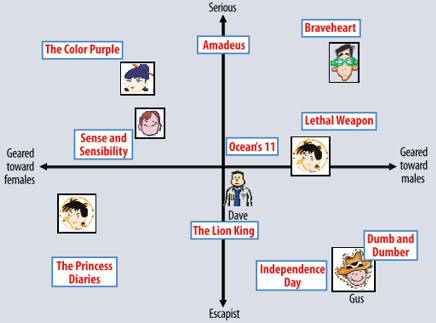【注：由于字数受限，题目中的RS代表Recommender Systems】

推荐系统中的常见的三大算法及各自优缺点

models，简称LFM），其中后两者统称为协同过滤算法（collaborative filtering，简CF）。下面是三者的关系示意图：

CBF通过给用户、物品定义显式的属性（通常会找所涉及的推荐领域的人类专家来定义）来描述他们的本质，然后为用户推荐与他们本质“门当户对”的物品；CF则是通过发动“群体的力量”，从其他用户、物品中学习到宝贵的信息，无需显式地定义属性：CF下的邻域算法着重于学习用户与用户、物品与物品之间的关系，为目标用户推荐与目标用户相似的用户所选择的物品（user-based）或者与目标用户所选择的物品相似的物品（item-based）；CF下的隐语义模型则是通过学习用户与用户、物品与物品之间的关系来自动获得用户、物品的隐属性（这里的“隐”指的是学习到的属性是不可解释的），相当于把用户-评分矩阵分解成用户隐属性矩阵和物品隐属性矩阵，然后通过用户隐属性向量u与物品隐属性向量i作点乘来获取到该用户对该物品的评分，以此为依据进行推荐。下面的表格列出了三种主流方法优缺点：

矩阵分解过程

def sgd(data_matrix, user, item, alpha, lam, iter_num):

for j in range(iter_num):
for u in range(data_matrix.shape):
for i in range(data_matrix.shape):
if data_matrix[u][i] != 0:
e_ui = data_matrix[u][i] - sum(user[u,:] * item[i,:])
user[u,:] += alpha * (e_ui * item[i,:] - lam * user[u,:])
item[i,:] += alpha * (e_ui * user[u,:] - lam * item[i,:])
return user, item

def sgd_bias(data_matrix, user, item, alpha, lam, iter_num, miu):

b_u =  * rating_matrix.shape
b_i =  * rating_matrix.shape
for j in range(iter_num):
for u in range(data_matrix.shape):
for i in range(data_matrix.shape):
if data_matrix[u][i] != 0:
b_ui = b_u[u] + b_i[i] + miu
e_ui = data_matrix[u][i] - b_ui - sum(user[u,:] * item[i,:])
user[u,:] += alpha * (e_ui * item[i,:] - lam * user[u,:])
item[i,:] += alpha * (e_ui * user[u,:] - lam * item[i,:])
b_u[u] += alpha * (e_ui - lam * b_u[u])
b_i[i] += alpha * (e_ui - lam * b_i[i])
return user, item, b_u, b_i